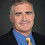## Wednesday, September 04, 2002

### Complexity Class of the Week: PP

Previous CCW

A language L is in PP if there is a nondeterministic polynomial-time Turing machine M such that x is in L if and only if M(x) has more accepting than rejecting paths.

PP stands for "Probabilistic Polynomial-time" from the original definition by Gill where one uses a probabilistic poly-time TM where x is in L if and only if the probability of M(x) accepting is greater than 1/2. "Probabilistic Polynomial-Time" would be a better name for PP's cousin class BPP or "Bounded-error Probabilistic Polynomial-Time". PP is not much use as a probabilistic class since it would take potentially an exponential number of trials to distinguish accepting from rejecting with reasonable confidence

A better name for PP would be Majority-P as given by the definition above. Because of historic reasons we are stuck with the name PP for now. Don't hold the bad name against the class. It still has a natural definition and some amazing properties.

PP has similar complexity to the function class #P as PPP = P#P, proved using the old stalwart, binary search. I have never found the paper that first proves this equivalence. Toda's Theorem shows the amazing hardness of PP by reducing the polynomial-time hierarchy to PPP.

Valiant's proof that the permanent is #P-complete also gives a natural complete language for PP:

PERM = { (M,k) | The permanent of M is at least k}

NP is in PP by adding a larger number of dummy accepting paths. PP is clearly closed under complement so we have co-NP in PP. Beigel, Reingold and Spielman show that PP is closed under union, a far trickier proof than one would expect using the fact that rational functions approximate the sign function well. Fortnow and Reingold build on their techniques to show that PP is closed under truth-table reductions.

PP is the prototypical counting class, classes defined in terms of the number of accepting and rejecting paths. PP contains the counting class C=P where x is in the language if the number of accepting paths equals the number of rejecting paths. On the other hand there are relativized worlds where ⊕P and PP are incomparable.

The limitations of PP come from what I call the Beigel all-purpose oracle. Beigel exhibits a relativized world where PNP is not contained in PP. His proof works by showing that any polynomial whose sign is the ODDMAXBIT function must either have high-degree or very large coefficients.

PP has interesting relationships to other complexity classes. Vereshchagin shows that Merlin-Arthur games, the class MA, is contained in PP but gives a relativized world where AM is not in PP. Adleman, DeMarrais and Huang show that the quantum class BQP is contained in PP. Watrous shows that QMA, the quantum version of MA, is also contained in PP.

Fortnow and Rogers, building Lide Li's Ph.D. thesis, show that BQP is low for PP, i.e., PPBQP = PP. Köbler, Schöning and Torán also using the work of Li show that graph isomorphism is also low for PP.

Allender shows that PP is not equal to uniform-TC0, constant-depth circuits with threshold gates. Can one show that PP is not equal to log-space? All one has to do is show that Toda's theorem can be extended to get any nonconstant level of the polynomial-time hierarchy to fall in PPP.

1.How is it exactly that you prove P^PP = P^#P? You said binary search, but I cant make sense of that.

1.If f is a #P function then the language L={(x,k) | f(x) >= k} is in PP. Then do binary search on k.

2.That means that if you have an oracle for PP, you can find the value of f(x), by doing binary search on k with L and therefore P^#P is in P^PP, correct? What about the other way (P^PP is in P^#P)? Even if you have an oracle for #P, how can you tell if more than half of the computational paths are accepting paths for an arbitrary problem?

2.You don't talk about the result of Scott Aaronson:

"Post-BQP = PP".

And yet, some proofs of PP class in 'Quantum Computing'.

My first comments here ... Awesome & amazing blog, bye !!!!

3.Projected model counting is in PP^NP. I see that PP^BQP = PP was known in 2002, is there anything known about PP^NP now?